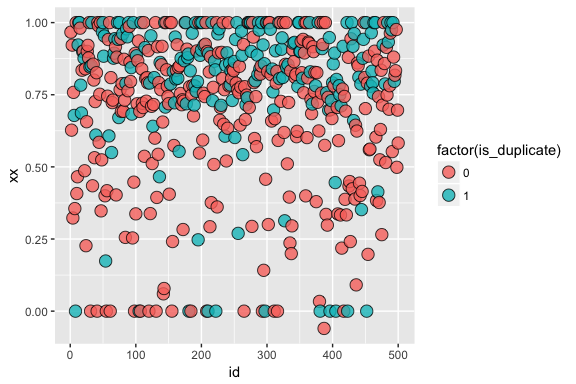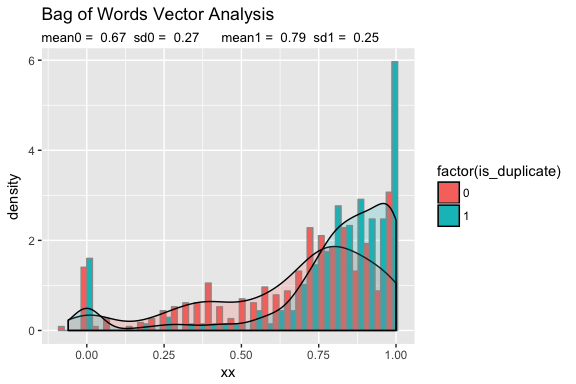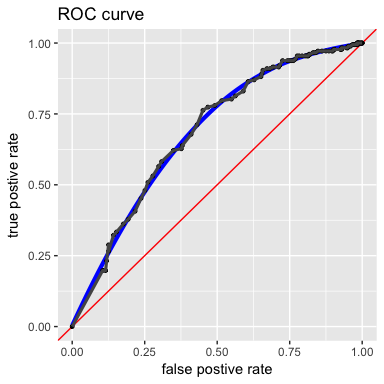Summary

A first pass analysis of the Quora Duplicate Questions dataset problem. A basic model using straight forward application of word vectors has an ability to disciminate duplicate sentences, with a measured postiive predictive value of about 51.77%.

This program uses these packages tidyverse, stringr, ggplot2, ww44ss/yo, stats, xtable, knitr, dplyr, purrr, broom, tidyr, tidytext
## Get The Data

Read the data as a .csv file. To make processing time sane, only the first 500 lines are processed

## define directory
data.directory <- "/Users/winstonsaunders/Documents/kaggle_quora/data/"
## read data into a data_frame
## fix the id
train.data <- train.data %>% mutate(id = id+1)

#test.data <- read_csv(str_c(data.directory, "test.csv"), col_types = "dcc")
## reduce size for prototyping

Data Cleaning

The purpose here is to put the data into a tidy format. The tools of the tidytext package accomplish this quickly.

First we get the list of stopwords from one of the supported dictionaries.

## GET STOP WORDS

list_of_stop_words <- stop_words %>%
filter(lexicon == "onix") %>%   #snowball
select(word) %>%
yo

# # A tibble: 174 × 1
#         word

Then quickly process the text into tidy format (yes, it is that easy!)

## TRANSFORM TRAINING DATA TO TIDY FORMAT

## Takes lightly processed train.data and reduced to single question, removes tokens,
## and gets rid of stop words

## QUESTION1
words_question1 <-
train.data %>%
select(id, qid1, question1) %>%
unnest_tokens(word, question1) %>%
filter(!word %in% list_of_stop_words$word) %>% yo xtable(words_question1[1:10, ]) % latex table generated in R 3.3.3 by xtable 1.8-2 package % Thu Apr 6 16:43:46 2017 ## the data should look like this # # A tibble: 1,700 × 3 # id qid1 word Let’s compare a couple of random questions with the data treated. ## print the reduced words id.select = sample(1:n.lines.read, 1) print(words_question1 %>% filter(id == id.select)) ## # A tibble: 3 × 3 ## id qid1 word ## <dbl> <dbl> <chr> ## 1 451 898 travel ## 2 451 898 faster ## 3 451 898 light print(words_question2%>% filter(id == id.select)) ## # A tibble: 3 × 3 ## id qid2 word ## <dbl> <dbl> <chr> ## 1 451 899 travel ## 2 451 899 faster ## 3 451 899 light ## print the reduced words id.select = sample(1:n.lines.read, 1) print(words_question1 %>% filter(id == id.select)) ## # A tibble: 3 × 3 ## id qid1 word ## <dbl> <dbl> <chr> ## 1 445 886 answer ## 2 445 886 question ## 3 445 886 quora print(words_question2%>% filter(id == id.select)) ## # A tibble: 3 × 3 ## id qid2 word ## <dbl> <dbl> <chr> ## 1 445 887 you've ## 2 445 887 learned ## 3 445 887 quora Vector Similiarity We can compute vector similarity by summing word vectors and taking the normalized dot product. Getting the word vectors The word vectors are predefined. We’ll use the 300 dimension GloVe word vectors from Stanford. I store a copy locally to speed things up. A bit of prcessing is required to get the vectors into a simple to use data_frame format. ## Grab word vectors number.of.words <- 9999 word.vector.file <- "glove.6B.300d.txt" directory.wordvecs <- "/Users/winstonsaunders/Documents/presidential_debates_clinton_saunders/" Processing the first 9999 of the GloVe word vectors glove.6B.300d.txt took 40 seconds to: 1. load the word vetors and 2. put them into usable form. Using the Word Vectors To use the word vectors we define a couple of utility functions vectorize.word <- function(word.x = "test", w.list = word.list){ ## compute normalized word vector ## inputs: ## word.x = sample word ## w.list = word.list data frame ## outputs: ## vec = a word vector vec <- word.x %>% paste0("^", ., "$") %>%
grep(w.list) %>%
slice(word.vector.df[ , -1], .) %>%
as_data_frame %>%
yo

return(vec)
}

After this it is a simple process to convert each question into a vector…

And then do a vector product comparison.

question.compare <- function(x){

dot.prod <- 0
if(x %in% 1:max(words_question2\$id)){

question1 <- words_question1 %>% filter(id == x) %>% select(word)
question2 <- words_question2 %>% filter(id == x) %>% select(word)

v1 <- question.2.vec(question1)
v2 <- question.2.vec(question2)

dot.prod <- sum(v1*v2)/sqrt((sum(v1*v1)*sum(v2*v2) + 0.001))
}

return(dot.prod)
}

Results

Visualization

The first way to look at the results is just to plot thee vector product of the two questions by question-pair id and color code the data based on the “grounded truth” know from the training data.Distribution

We can get a little better idea by taking a historgram of the data, again grouping by the grounded truth. This confirms what may be hard to recognize in the data above, namely that the model does indeed discriminate between types of questions (though the distributions do have considerable overlap).ROC Curve

Based on the observation above, we can forge ahead and compute a ROC curve.

##  254
##  172Here we can see some reasonable discrimination in true versus false positive.

The postive predictive value of this model is roughly 52%.

Conclusion

For a first pass, the word vector analysis shows some promise of having predictive value, especially it it can be combined with other predictors. The next steps are to explore refinement of and additions to the model.